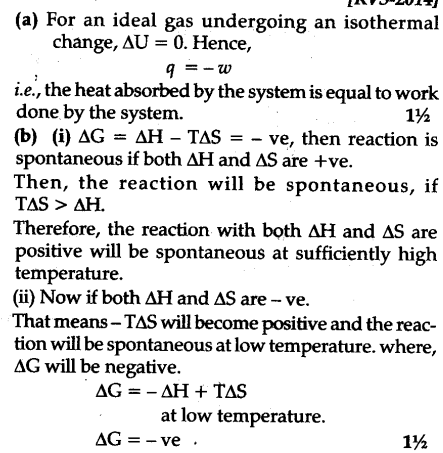# A) Under what conditions is the heat absorbed by a system is equal to the work done by the system

(a) Under what conditions is the heat absorbed by a system is equal to the work done by the system.
(b) Under what conditions the following reaction will be spontaneous:
(i) Reaction with both \$\Delta \$H and \$\Delta \$S positive.
(ii) Reaction with both \$\Delta \$H and \$\Delta \$S negative.